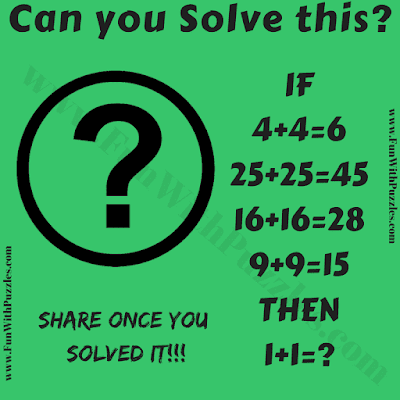It is very interesting Logic about Maths Numbers Problem. In this logic Maths Picture puzzle there are some Mathematical equations. These Maths Equations contain some logical pattern that you have to decode the find the value of the last equation. Can you solve this logic about Math Numbers Problem?Can you solve this logical Math Numbers Problem?

The answer to this "Logic about Math Numbers Problem", can be viewed by clicking on the button. Please do give your best try before looking at the answer.